# How to find out the amount from a period with conditions in Google SheetsWhen interacting with Google Sheets, the user can create records every day, indicating the date and some number next to it, for example, the amount of money earned. There may be another condition – the type of activity in which this amount was earned. Weeks or even months will pass, the table will fill up and you will need how much money was earned during a certain period in a certain area. You can calculate the required amount by two different methods, which I want to demonstrate clearly.

I would like to draw your attention to the fact that in the introductory paragraph I described only an example. There can be an unlimited number of practical methods of using the formulas described below. It all depends solely on your goals and initial data. The same applies to the number of conditions, because all formulas can be scaled, which will also be demonstrated, but at the end of the article, so as not to complicate the already not the simplest explanation.

## Initial data and preparatory work

I’ll take the table whose content I’ve already described briefly above, along with doing some prep work to optimize it and make it easier to calculate later. You can copy this example in its entirety or use it just to familiarize yourself with how the formulas work. Let’s start by listing the raw data:

1. In one column we have consecutive days, which will be a period in the future.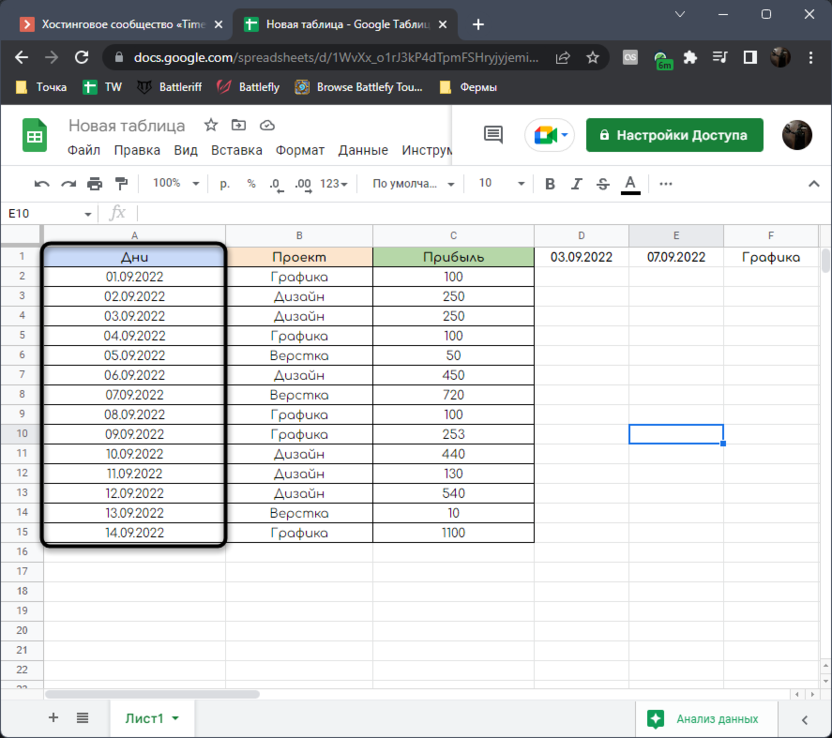2. The next column shows the field of activity that the user was engaged in on each of these days.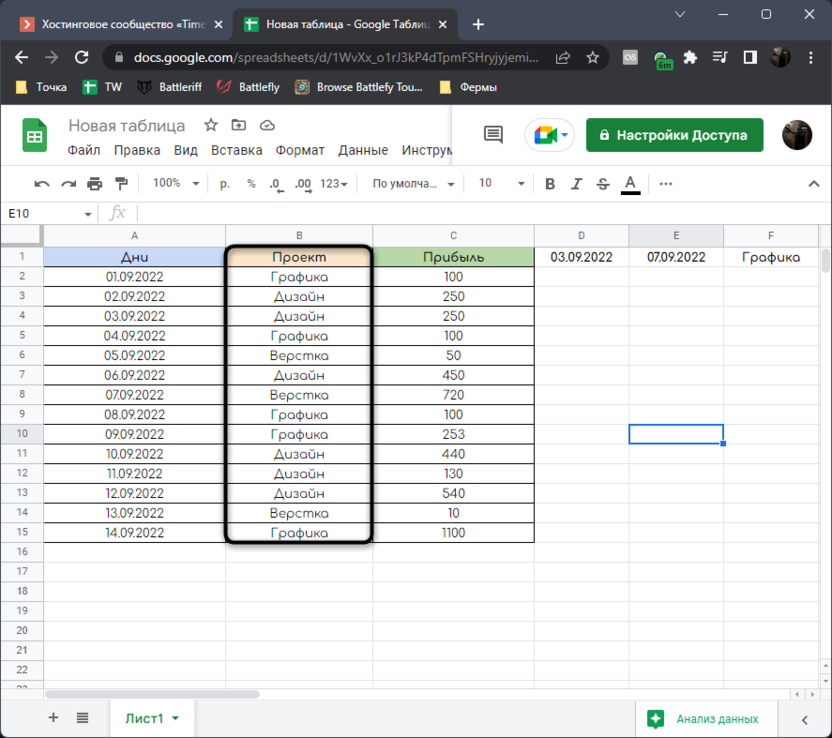3. The latter is the received profit, the amount of which will be calculated depending on the set period and additional conditions.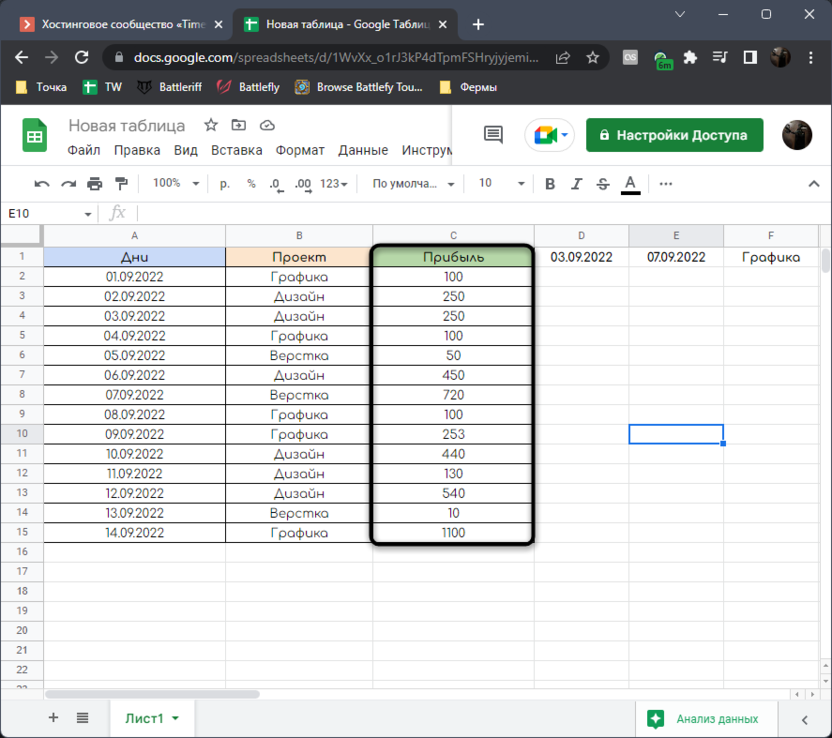4. In the lines above, I indicate the start date of the period, the end amount and the field of activity from which the earnings need to be found.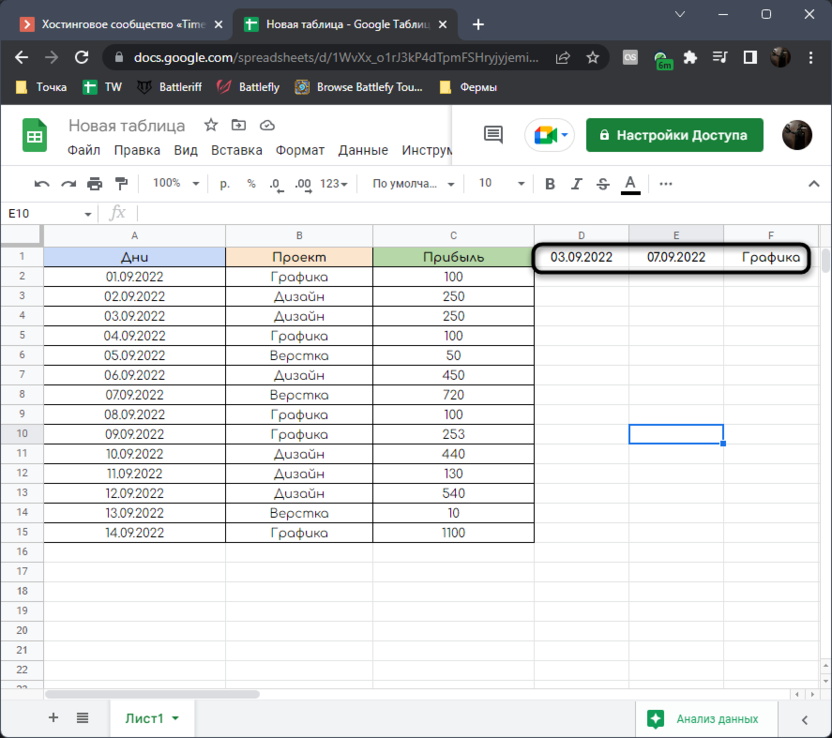You can use simple day numbers from one to infinity, a different date format, add multiple conditions, or sum other values ​​instead of profit. Everything depends only on the table you have in hand.

Now let’s quickly touch on the simple topic of preparatory work. I will use data validation to generate a list in these most helpful cells. This will help you not to edit them every time you change the period or conditions, but simply select the desired value from the list.

1. First, let’s deal with the initial date of the period. Select this same cell and expand the menu “Data”.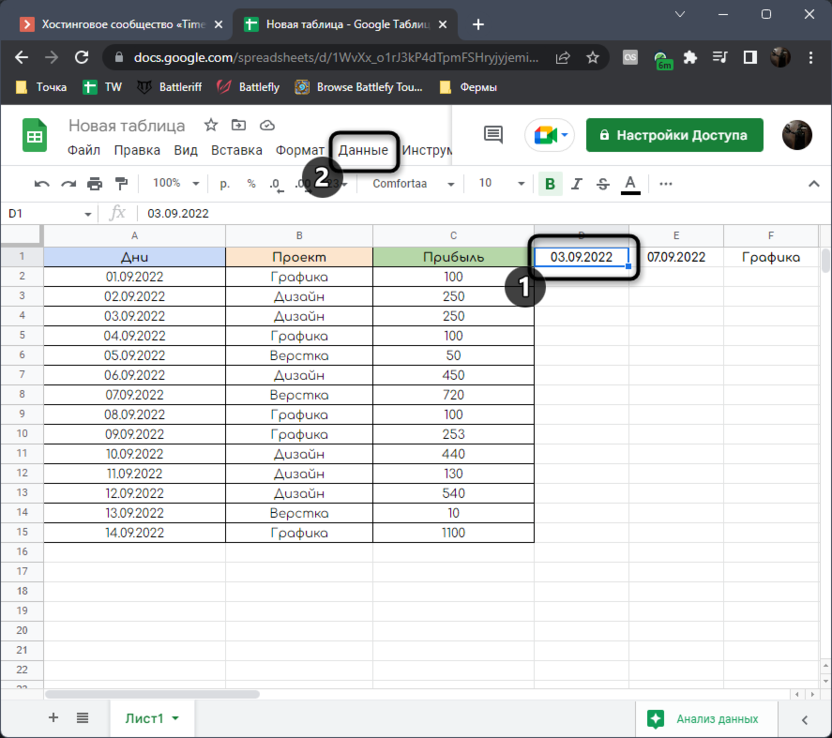2. Select an item from it “Configure Data Validation”.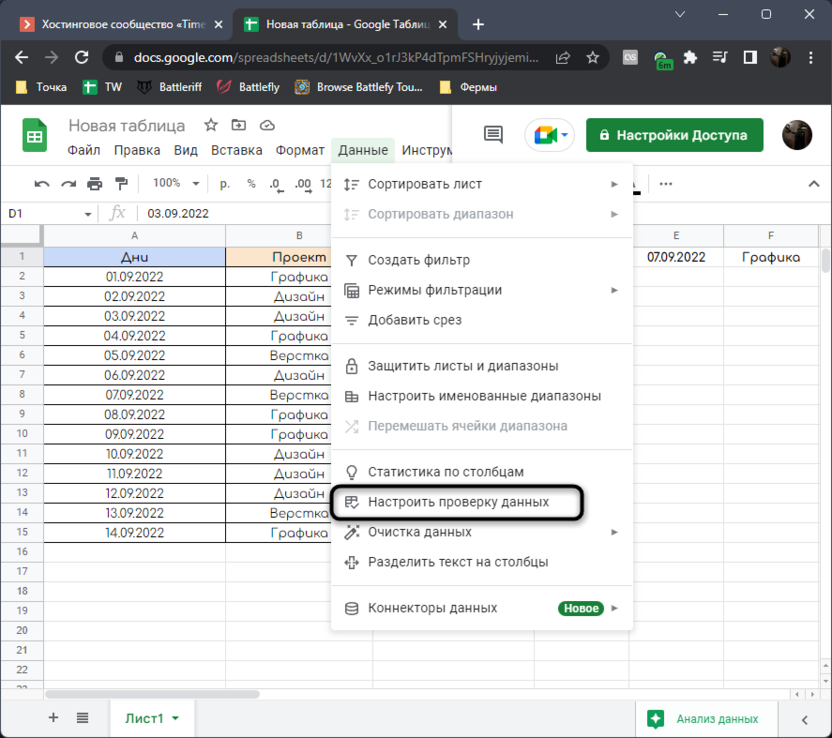3. You need to enter only one change – specify the column from which this data will be taken. Accordingly, highlight the range where all your numbers are written, and then save the changes.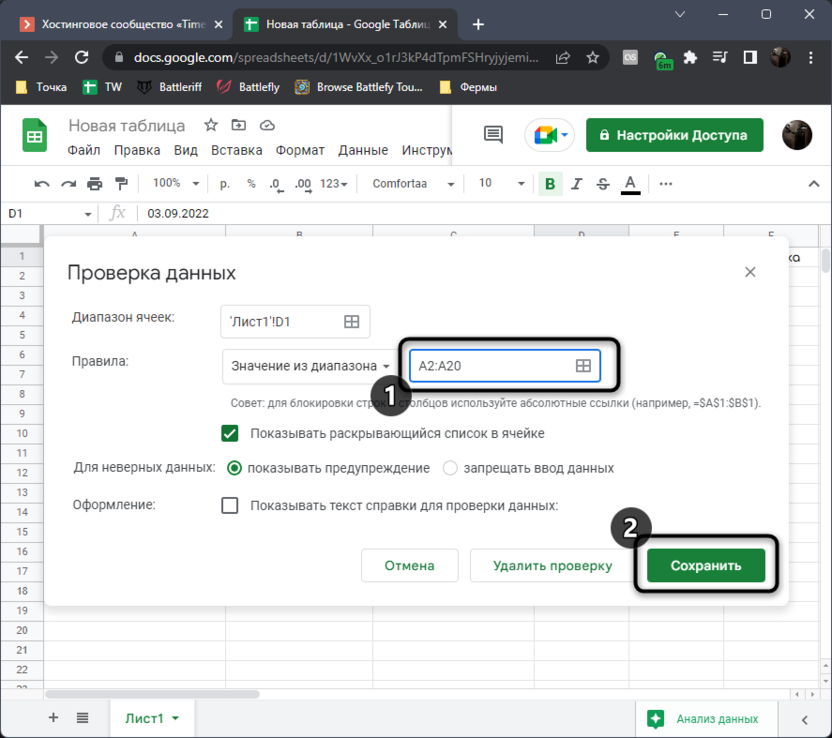4. A down arrow button will now appear next to the number.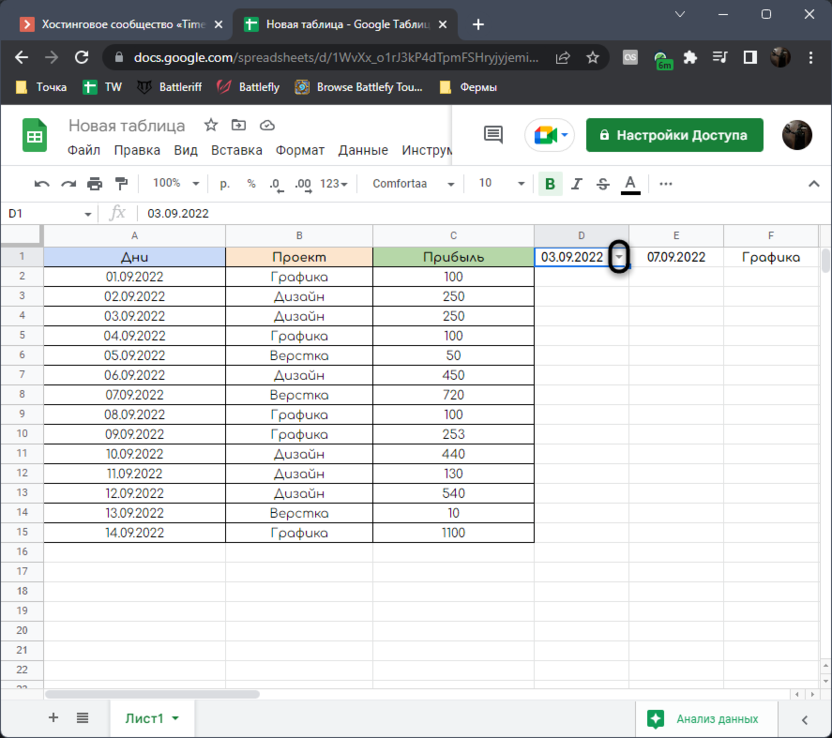5. Click on it to display a list of all dates. You can choose any one from it, which will mark the beginning of your period.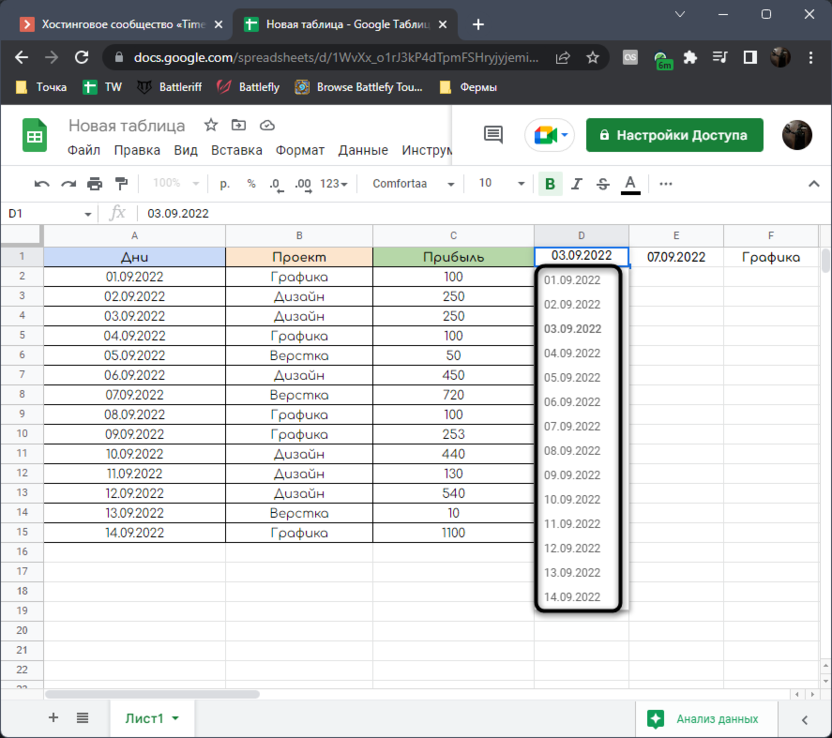6. Do exactly the same thing with the end date cell, so that you can also switch if necessary.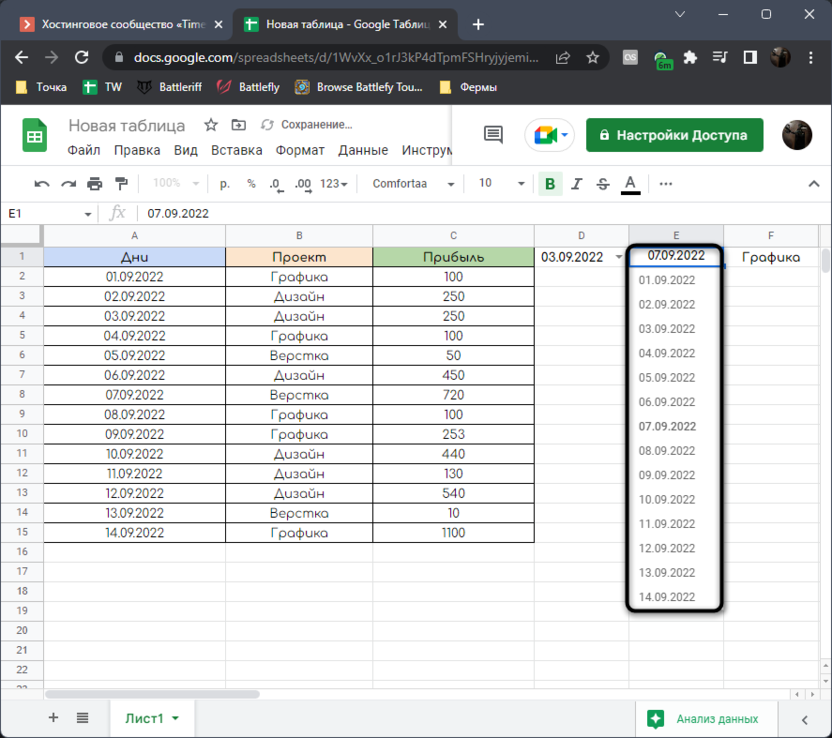7. With additional conditions, everything is about the same. Select an empty cell where you want to create a list, remember the column number and go to the menu “Data”.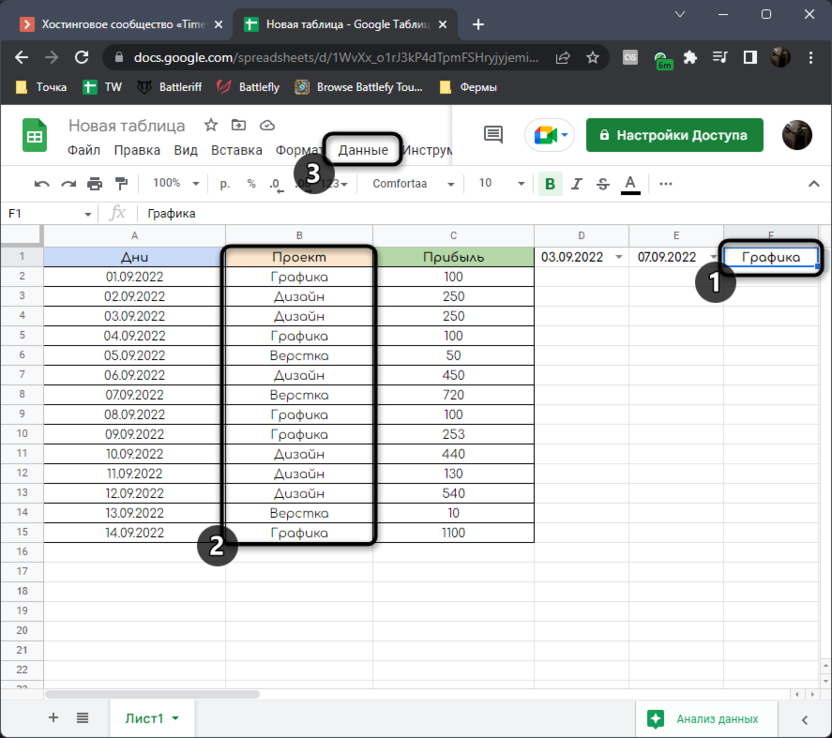8. Call the function again “Configure Data Validation” and in the new window, set the appropriate range in the same way as before.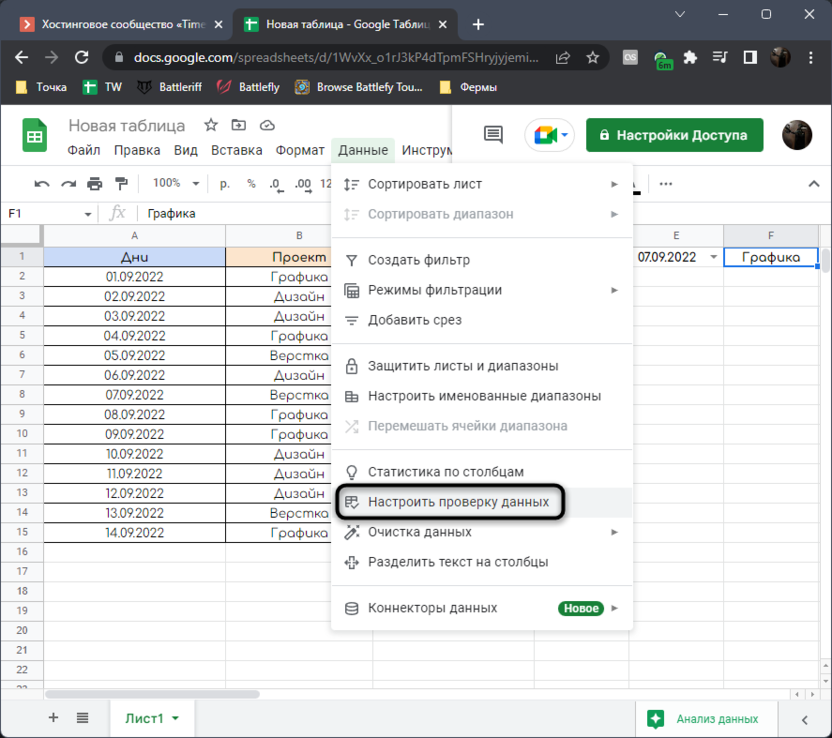9. The main thing is to write down the same areas of activity with the same symbols so that the list is formed correctly, without unnecessary duplicates.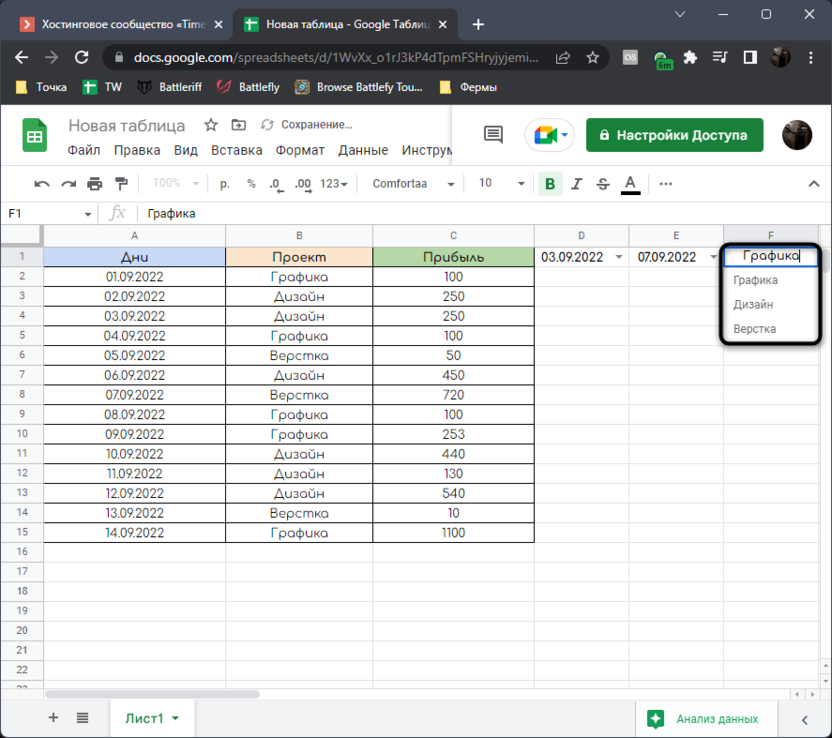This completes the familiarization with the table itself and the preparatory work, so let’s proceed to the analysis of the first, more complex formula for calculating the information we need.

The community is now in Telegram

Subscribe and stay up to date with the latest IT news

## Method 1: Using the AND and COMBINATION functions

Function AND in Google Sheets allows you to compare the inequality and display the value in the cell TRUTH or Liestarting from whether the inequality condition is appropriate. With this function, we will create an auxiliary column with which we will calculate all the days included in the required period. Let’s visually analyze all the components of the first formula, which will be needed for further calculations.

1. Select the first cell in the column that you want to make secondary. Declare a function in it =AND.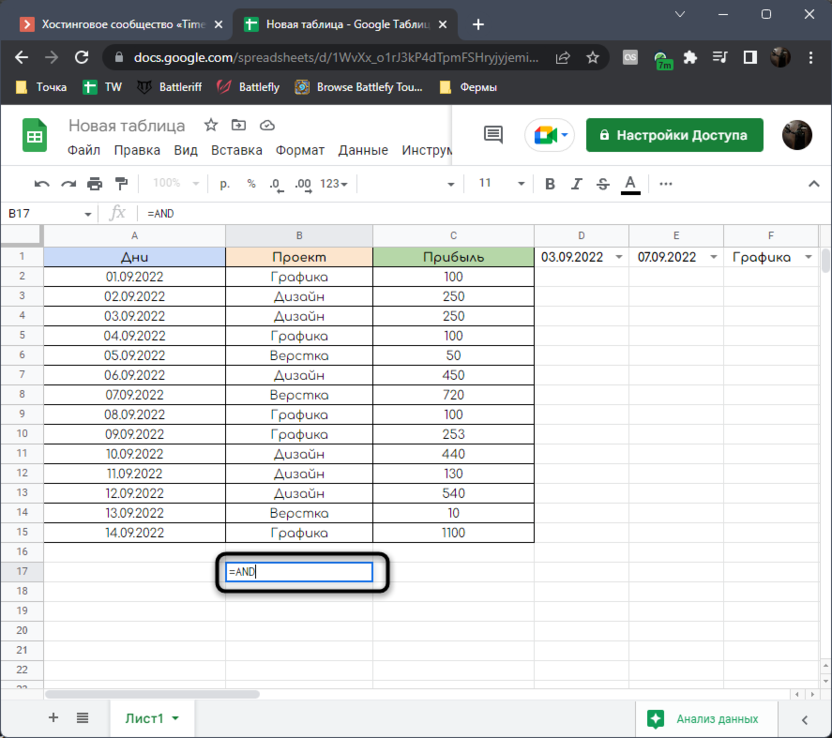2. Next, expand the brackets and specify the first condition of the inequality (A2>=\$D\$1;). Note that the cell D1 is static due to the addition of a constant, that is, it will not stretch when the column is further formed.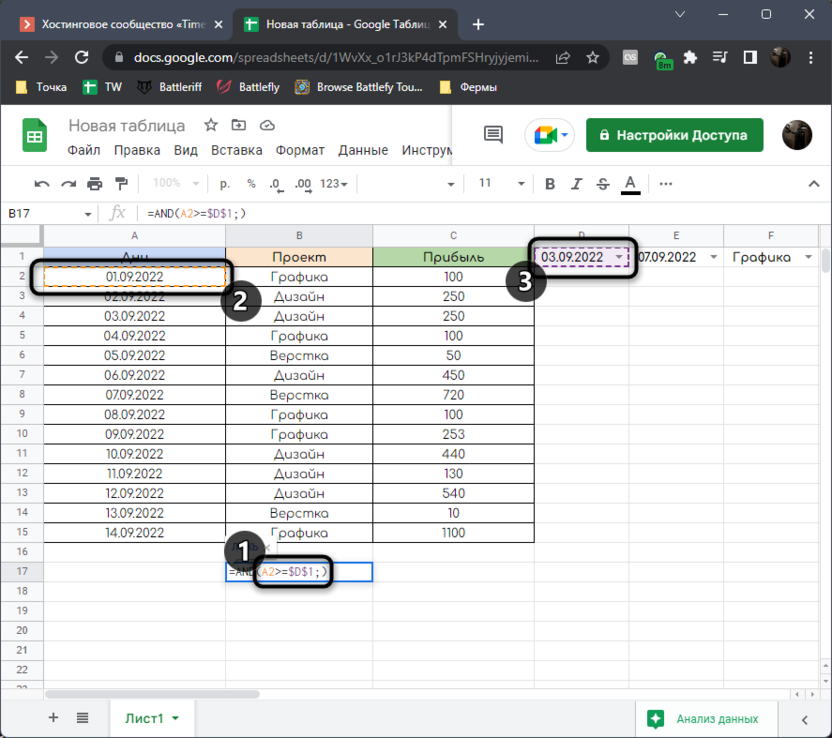3. The second condition A2<=\$E\$1where E1 – cell with the last date of the period.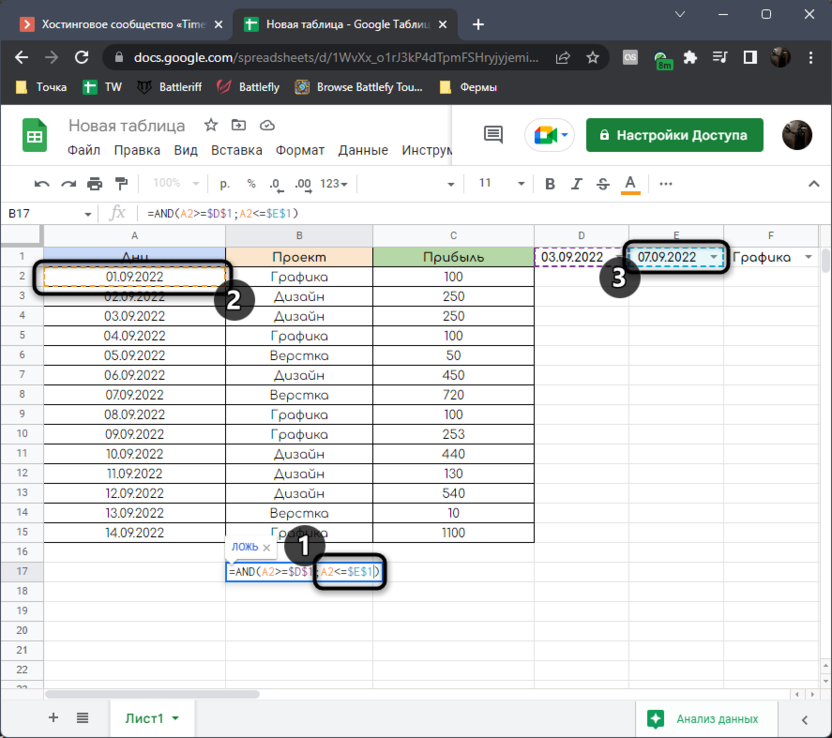4. Click Enter to confirm the formula and stretch it down as many cells as you have in the date column. As a result, it will look something like this: =AND(A2>=\$D\$1;A2<=\$E\$1;B2=\$F\$1).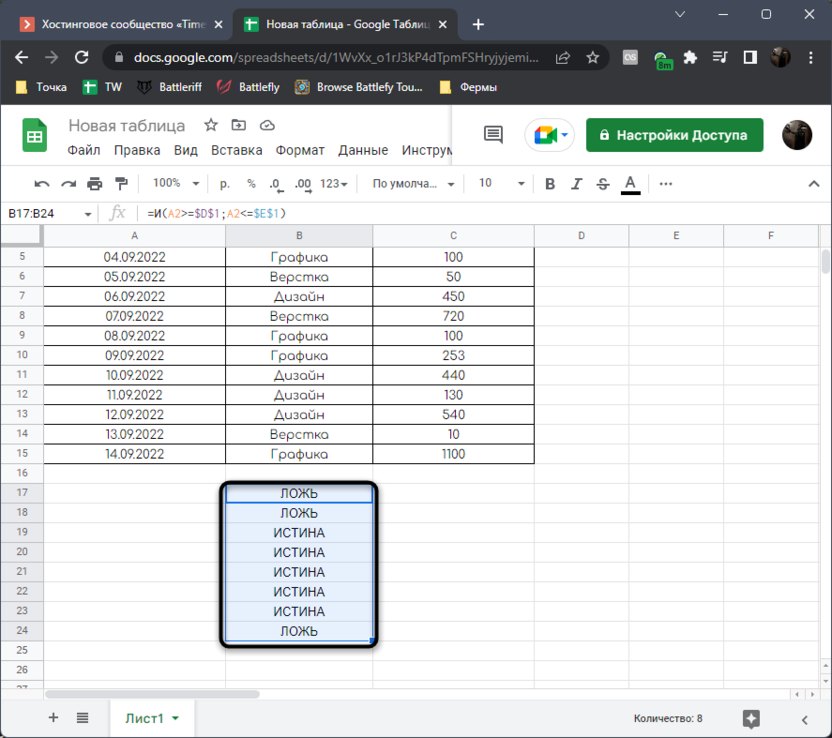With this function, we calculate the dates that are relevant for the user’s selected period. Value TRUTH or Lie will change depending on which dates you specify yourself as the beginning and end of the period. Accordingly, if the value TRUTH, So the date is included in the period and the profit value will be included in the formula. If Liethe cell is skipped and the transition to the next is made.

The first part of the formula is finished, all that remains is to create the basic calculations, which will include the values TRUTHS and Lies from the auxiliary column. This will help us CONSISTENT. This function evaluates only if the given condition is satisfied. Writing this formula in our case looks like this:

1. Declare a function =REASONABLE where you want to place the result of profit summation or other data calculated in the period.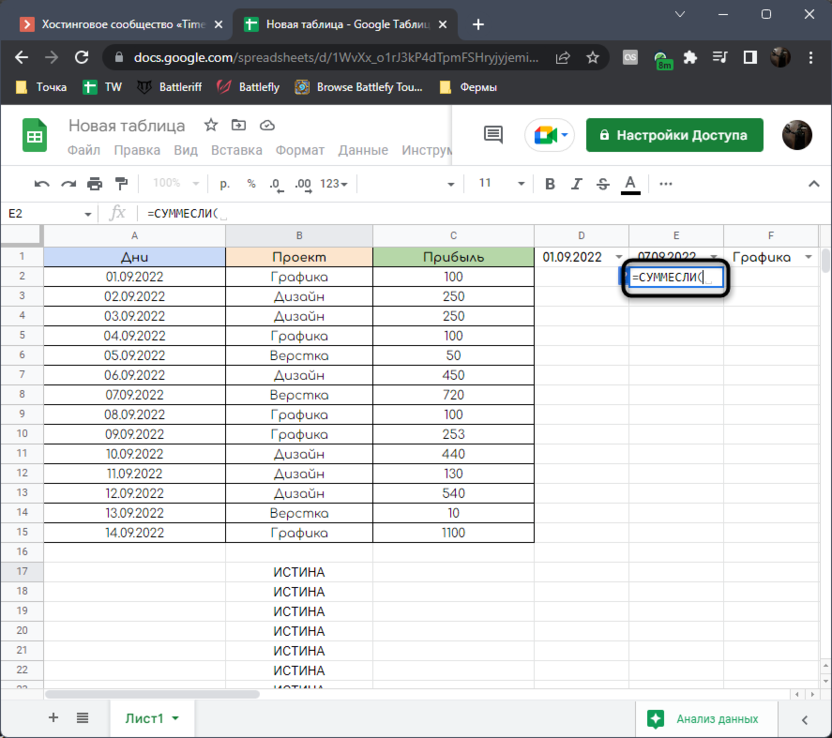2. As the first column to check, specify all cells with TRUTH and Liewhich we formed earlier.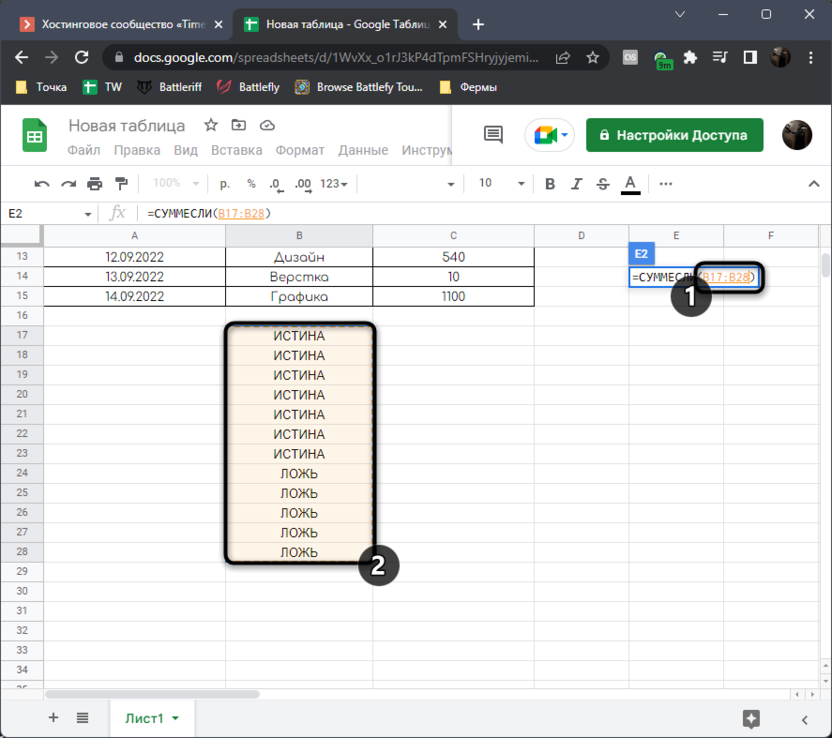3. Put a semicolon, add TRUTH, put a semicolon again and specify a data range with values ​​whose sum should be calculated. Click Enter and look at the result.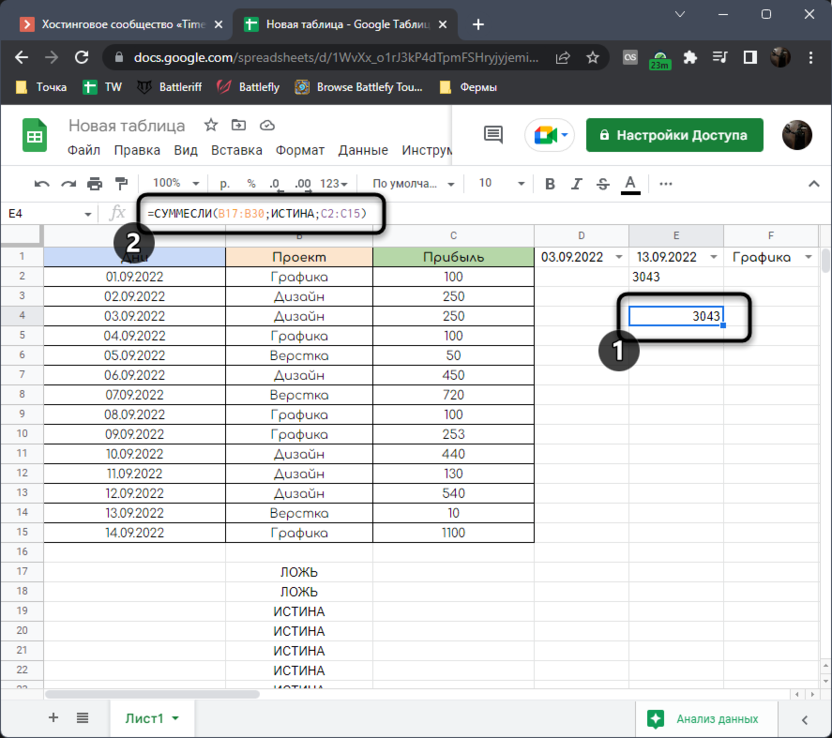4. I manually select all the cells that fall within the period each day and see that the formula works correctly.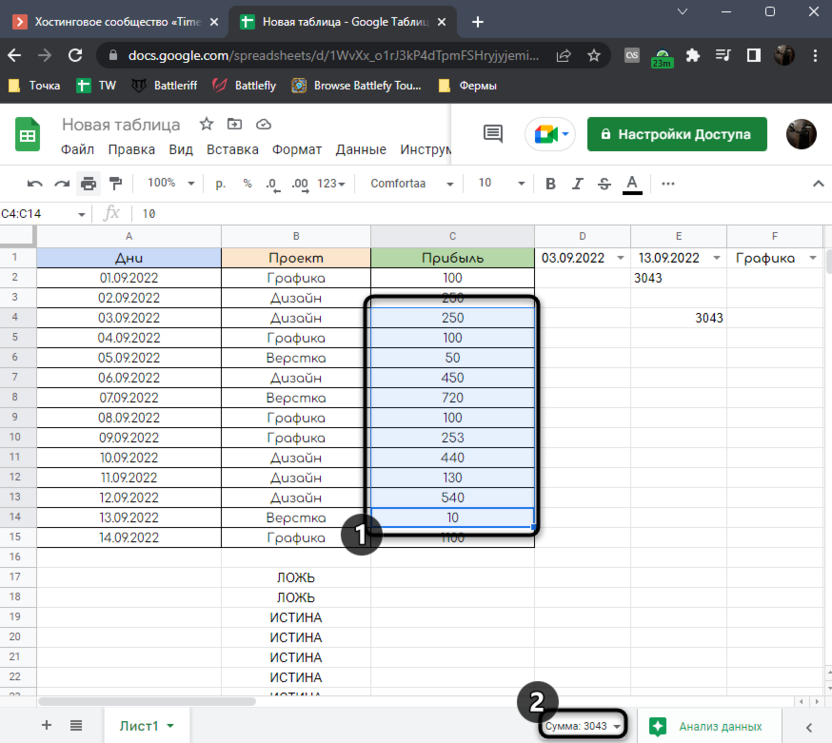Let’s visually analyze it. The formula looks like =COMPATIBLE(B17:B30;TRUE;C2:C15). First of all, the range to be checked is indicated, then the condition that suits us, that is, it should be TRUTH. The last argument is the data that will be summed if one TRUTHthat is, the column with our profit.

## Method 2: Using the SUMPRODUCT function

The previous method has one significant drawback – the need to use an auxiliary column and combine several functions. Yes, this column can be hidden or moved to another sheet, but this is not always appropriate. If you also think that the first method is not very suitable, let’s figure out how to perform this task, but using the function SUMPRODUCT.

Explaining the function itself is a bit more difficult. We give it several arguments with logical conditions, after which we indicate that it is necessary to sum up those values ​​that fall under the given conditions. Visually, if we are talking about solving today’s task, the use of this function looks like this:

1. Announce first =SUMPRODUCT or =SUMP PROVINCE in Russian, in any convenient cell where you want to display the result.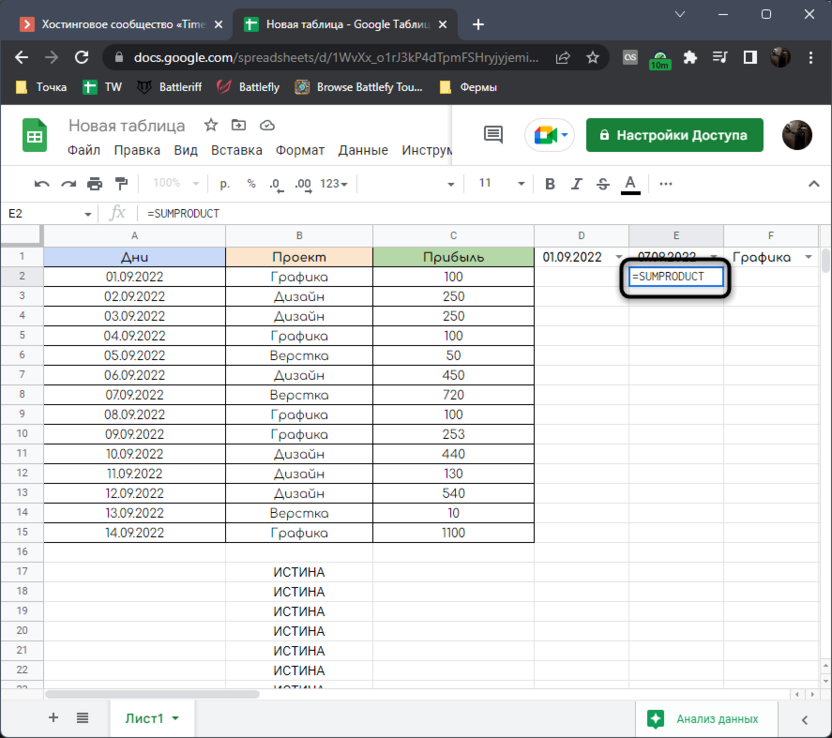2. Open the quotes and specify that the number range from the date range must be greater than or equal to the first number in the period. This is done in the same way as when creating an auxiliary column in Methods 1.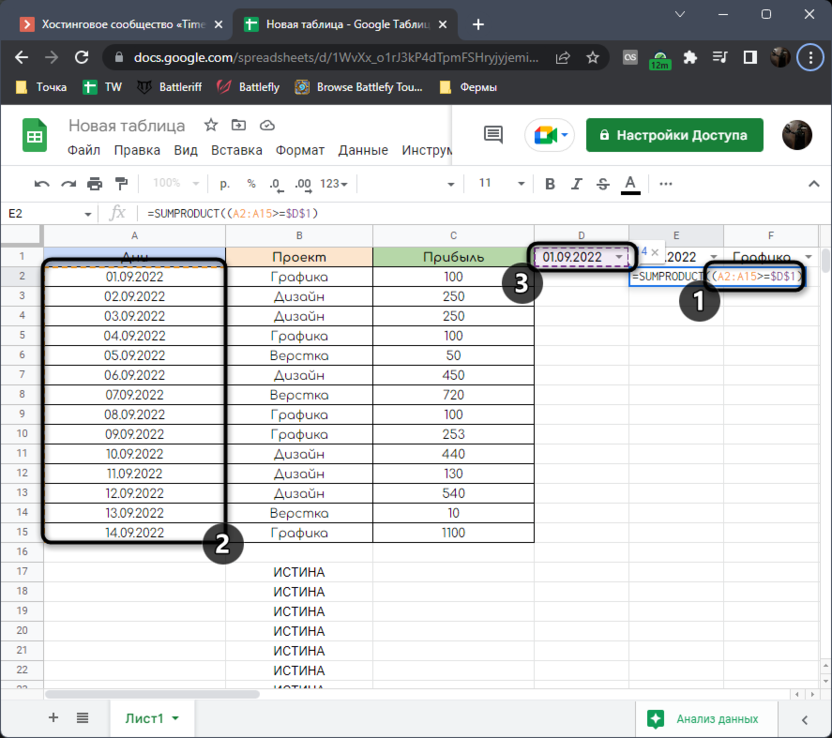3. Close the parentheses after the first condition and put a sign *that is, in our case, we will combine the previous condition with the following one. Just open the parentheses and write the second condition, specifying that the values ​​in the date range must be less than or equal to the last number in the period.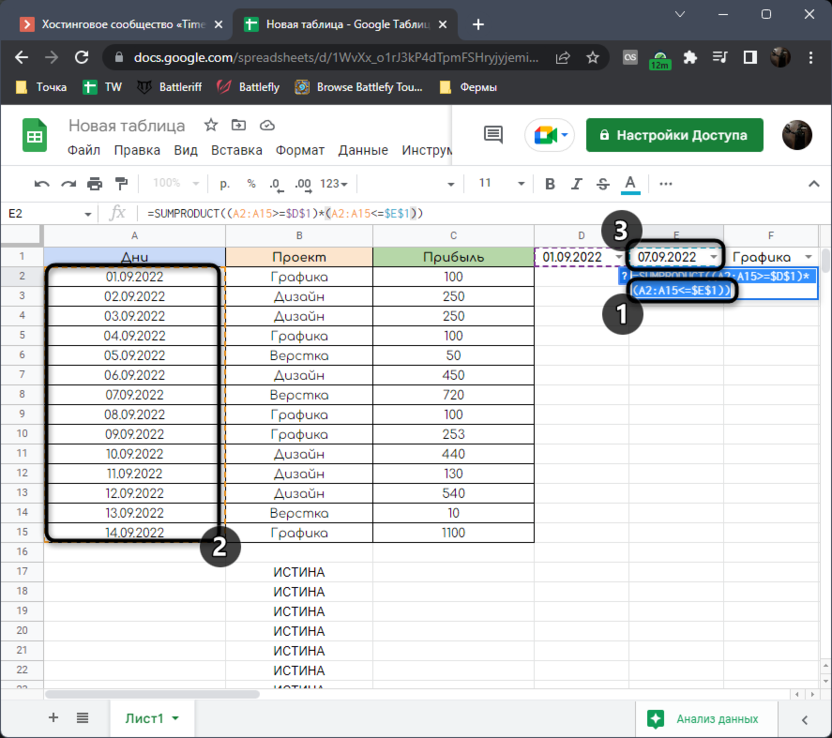4. It remains only to add one more sign * and add that it is necessary to sum up the numbers from the range with profit from the true values ​​of the conditions.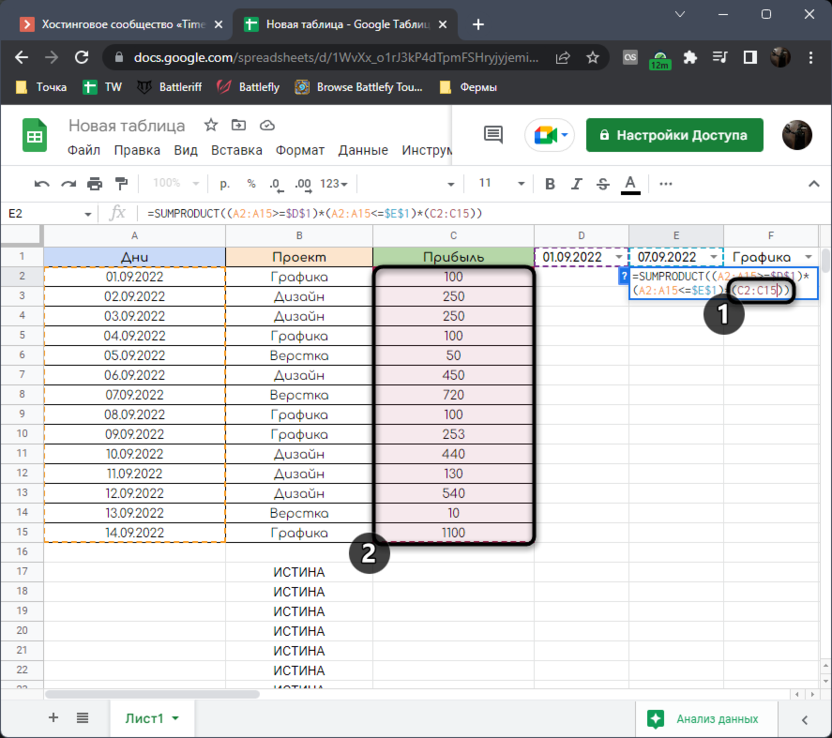5. Click Enter and check if the function worked. If not, read my instructions again and analyze its components with yours.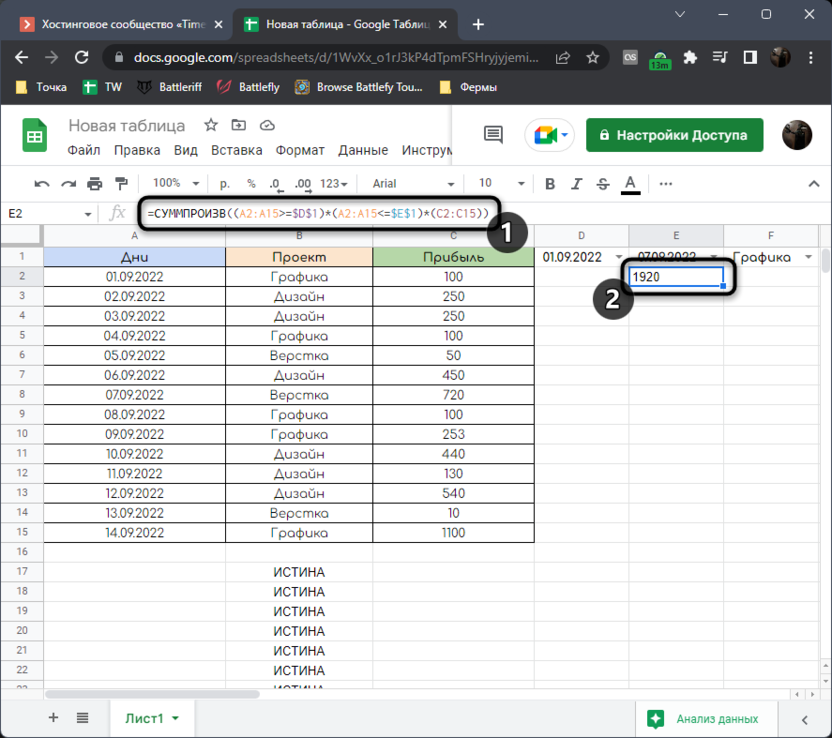The complete line with this formula looks like =SUMCALL((A2:A15>=\$D\$1)*(A2:A15<=\$E\$1)*(C2:C15)), so you can just copy and paste it if the cell numbers in the columns match. In this line, we’ve declared two boolean conditions, then told the formula what data to sum if it fits the given parameters.

## Adding conditions to a period

As I said, finally we will analyze the scaling of our formulas by adding various conditions to them. In my case, this is a type of activity that a person was engaged in every day and received income for it.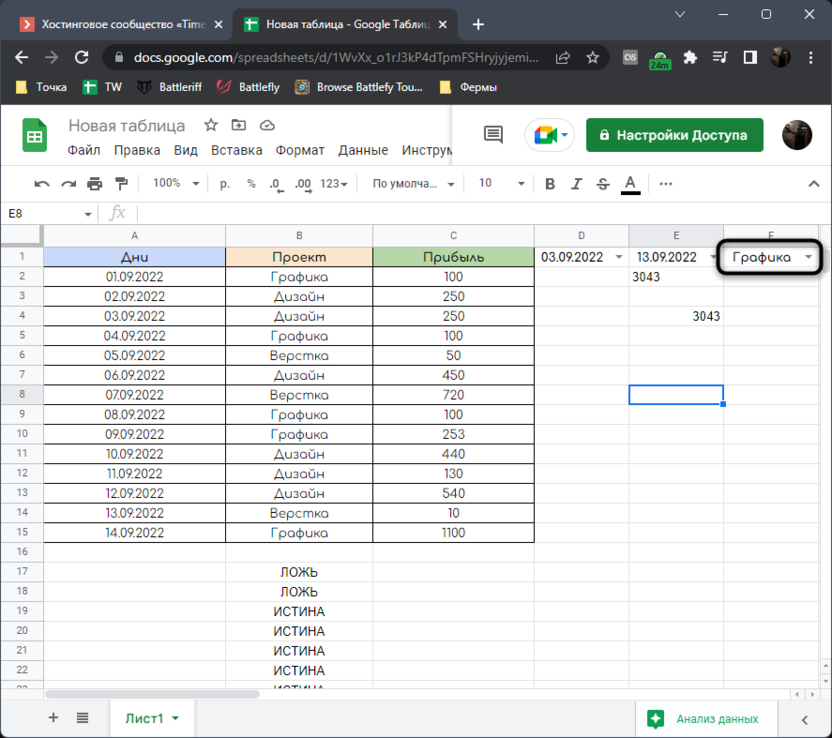It turns out that with the help of the formulas described above, it is necessary to calculate the amount not only in the given range, but also taking into account the type of activity recorded in the column next to it. Each of the formulas will have to be modernized a little, so let’s start with the first one.

1. Activate the first cell from the auxiliary column with a function AND and add another condition to it that looks like B2=\$F\$1. B2 – the first cell with the type of activity, a F1 – the same condition that we choose during calculations. It must be fixed so that the cell does not move further.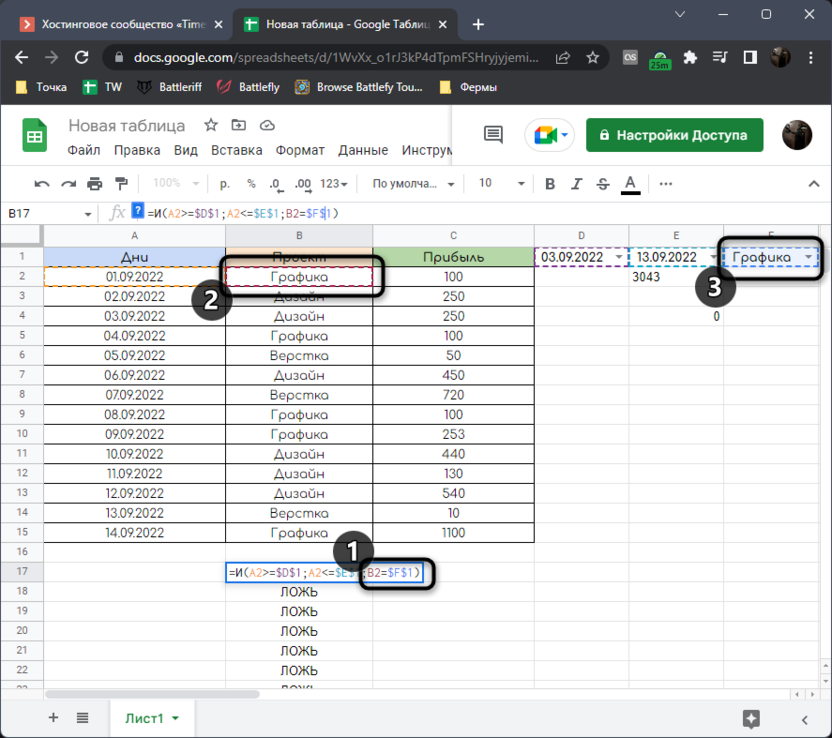2. Stretch the formula itself to the last cell of the auxiliary column by holding down the lower right corner with the left mouse button.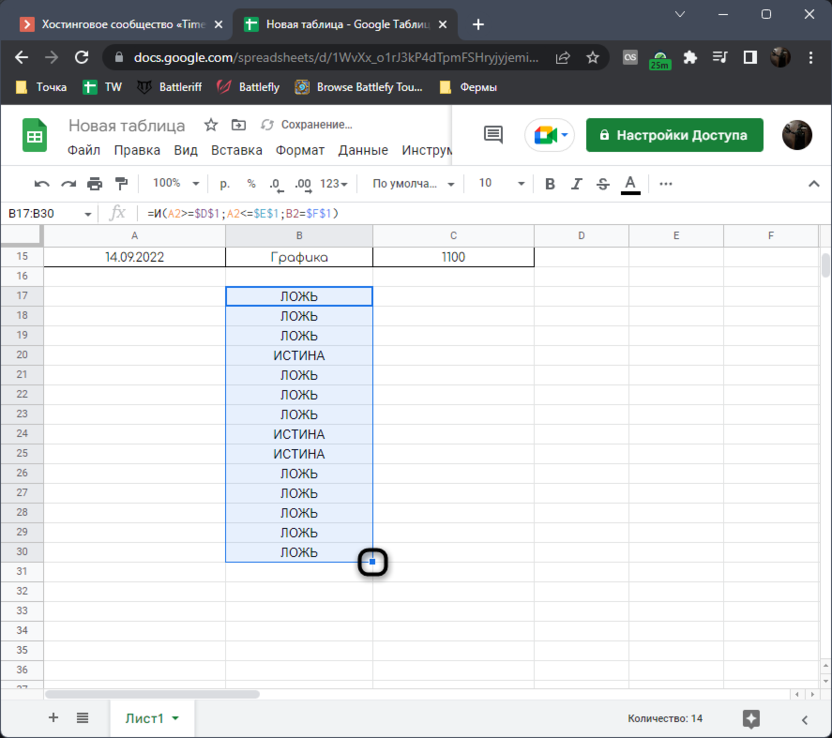3. In the function =REASONABLE changes will happen automatically as it takes data from TRUTH and Lie. Now you see that the formula calculates in a new way, taking into account the entered condition. There can be an almost unlimited number of such conditions.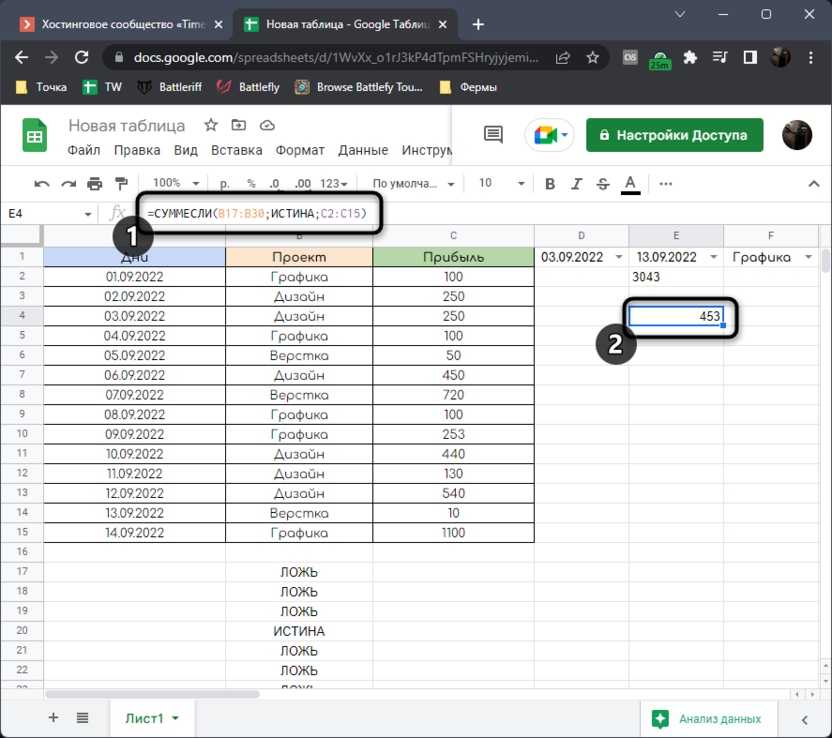If necessary, I leave the modernized function with the addition of one condition.

```
=AND(A2>=\$D\$1;A2<=\$E\$1;B2=\$F\$1)```

Approximately the same is done with the formula SUMPRODUCTwhere after two logical conditions you need to add a third, selecting the entire range with conditions.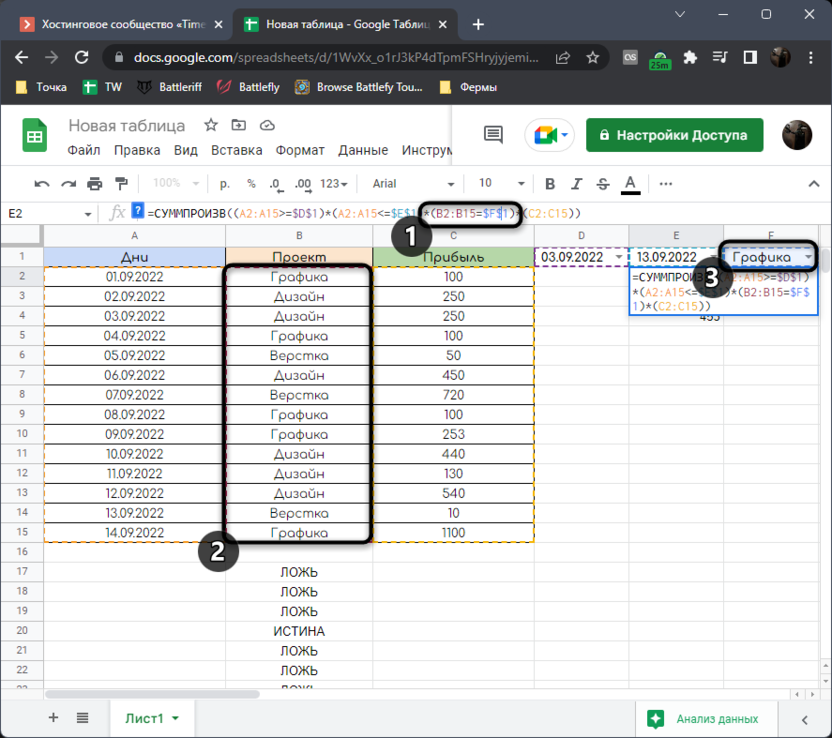The result is the same. At first, you can get confused in such a long line, but if you look at the highlighted columns and correctly determine the sequence of actions, everything becomes clear.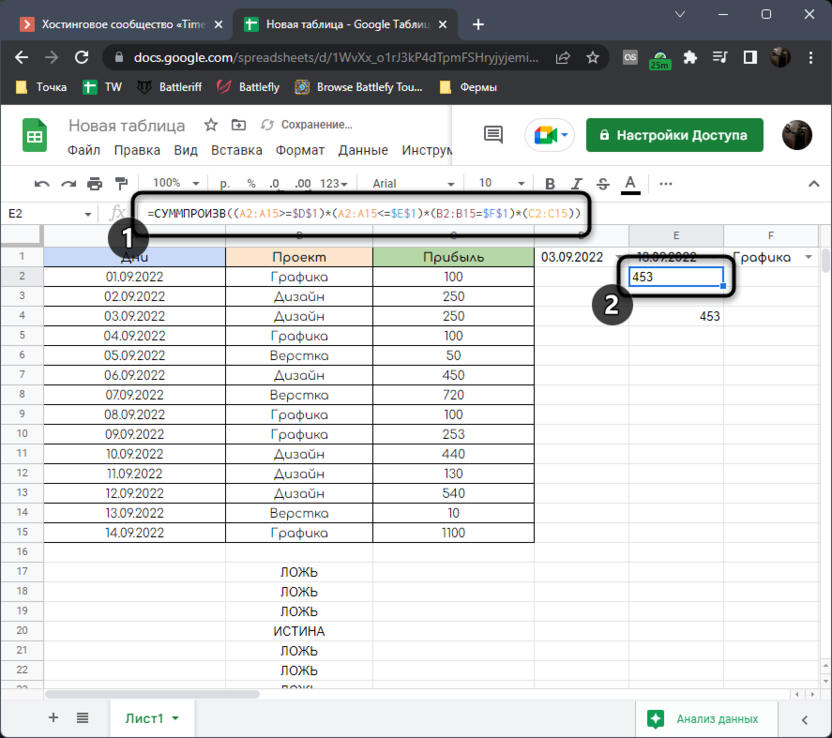As in the case of the previous formula, I provide a modernized version if you suddenly decide to copy it for future use.

```
=СУММПРОИЗВ((A2:A15>=\$D\$1)*(A2:A15<=\$E\$1)*(B2:B15=\$F\$1)*(C2:C15))```

You and I have dealt with the performance of not the easiest task. You learned that you can dynamically change the period for calculations and add different conditions. If necessary, combine the obtained results with other formulas, create pivot tables and much more. If you have any questions about the topic, feel free to ask them in the comments, and I will try to provide a prompt answer.